# 234 Results

View
Selected filters:
• Calculus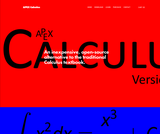Conditional Remix & Share Permitted
CC BY-NC
Rating

APEX Calculus is a calculus textbook written for traditional college/university calculus courses. It has the look and feel of the calculus book you likely use right now (Stewart, Thomas & Finney, etc.). The explanations of new concepts is clear, written for someone who does not yet know calculus. Each section ends with an exercise set with ample problems to practice & test skills (odd answers are in the back).

Subject:
Calculus
Material Type:
Textbook
Provider:
APEX: Affordable Print and Electronic TeXtbooks
Author:
Brian Heinold
Dimplekumar Chalishajar
Gregory Hartman
Troy Siemers
01/01/2014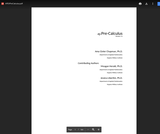Conditional Remix & Share Permitted
CC BY-NC
Rating

This text was written as a prequel to the APEXCalculus series, a three–volume series on Calculus. This text is not intended to fully prepare students with all of the mathematical knowledge they need to tackle Calculus, rather it is designed to review mathematical concepts that are often stumbling blocks in the Calculus sequence. It starts basic and builds to more complex topics. This text is written so that each section and topic largely stands on its own, making it a good resource for students in Calculus who are struggling with the supporting mathemathics found in Calculus courses. The topics were chosen based on experience; several instructors in the Applied Mathemathics Department at the Virginia Military Institute (VMI) compiled a list of topics that Calculus students commonly struggle with, giving the focus of this text. This allows for a more focused approach; at first glance one of the obvious differences from a standard Pre-Calculus text is its size.

Subject:
Calculus
Material Type:
Textbook
Author:
Amy Givler Chapman
Jessica Libertini
Meagan Herald
04/27/2020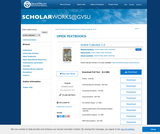Conditional Remix & Share Permitted
CC BY-NC-SA
Rating

Active Calculus is different from most existing calculus texts in at least the following ways: the text is free for download by students and instructors in .pdf format; in the electronic format, graphics are in full color and there are live html links to java applets; the text is open source, and interested instructors can gain access to the original source files upon request; the style of the text requires students to be active learners — there are very few worked examples in the text, with there instead being 3-4 activities per section that engage students in connecting ideas, solving problems, and developing understanding of key calculus concepts; each section begins with motivating questions, a brief introduction, and a preview activity, all of which are designed to be read and completed prior to class; the exercises are few in number and challenging in nature.

Subject:
Mathematics
Calculus
Material Type:
Textbook
Provider:
Grand Valley State University
Author:
David Austin
Matt Boelkins
Steve Schlicker
04/27/2020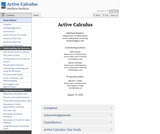Conditional Remix & Share Permitted
CC BY-NC-SA
Rating

Active Calculus is different from most existing calculus texts in at least the following ways: the text is freely readable online in HTML format and is also available for in PDF; in the electronic format, graphics are in full color and there are live links to java applets; version 2.0 now contains WeBWorK exercises in each chapter, which are fully interactive in the HTML format and included in print in the PDF; the text is open source, and interested users can gain access to the original source files on GitHub; the style of the text requires students to be active learners — there are very few worked examples in the text, with there instead being 3-4 activities per section that engage students in connecting ideas, solving problems, and developing understanding of key calculus concepts; each section begins with motivating questions, a brief introduction, and a preview activity, all of which are designed to be read and completed prior to class; following the WeBWorK exercises in each section, there are several challenging problems that require students to connect key ideas and write to communicate their understanding.

Subject:
Calculus
Material Type:
Textbook
Provider:
Grand Valley State University
Author:
David Austin
Matt Boelkins
Steve Schlicker
11/09/2021Conditional Remix & Share Permitted
CC BY-NC-SA
Rating

Active Calculus Multivariable is the continuation of Active Calculus to multivariable functions. The Active Calculus texts are different from most existing calculus texts in at least the following ways: the texts are free for download by students and instructors in .pdf format; in the electronic format, graphics are in full color and there are live html links to java applets; the texts are open source, and interested instructors can gain access to the original source files upon request; the style of the texts requires students to be active learners — there are very few worked examples in the texts, with there instead being 3-4 activities per section that engage students in connecting ideas, solving problems, and developing understanding of key calculus concepts; each section begins with motivating questions, a brief introduction, and a preview activity, all of which are designed to be read and completed prior to class; the exercises are few in number and challenging in nature.

Subject:
Calculus
Material Type:
Textbook
Provider:
Grand Valley State University
Author:
David Austin
Matthew Boelkins
Steven Schlicker
07/01/2018Conditional Remix & Share Permitted
CC BY-NC-SA
Rating

Published in 1991 by Wellesley-Cambridge Press, the book is a useful resource for educators and self-learners alike. It is well organized, covers single variable and multivariable calculus in depth, and is rich with applications.

In addition to the Textbook, there is also an online Instructor's Manual and a student Study Guide. Prof. Strang has also developed a related series of videos, Highlights of Calculus, on the basic ideas of calculus.

Subject:
Mathematics
Calculus
Material Type:
Full Course
Provider:
MIT
Author:
Gilbert Strang
01/01/1991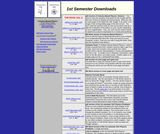Conditional Remix & Share Permitted
CC BY-SA
Rating

Calculus-Based Physics is an introductory physics textbook designed for use in the two-semester introductory physics course typically taken by science and engineering students.

Subject:
Calculus
Physics
Material Type:
Textbook
Provider:
Saint Anselm College
Author:
Jeffrey Schnick
04/27/2020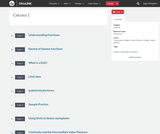Rating

The Calculus I course was developed through the Ohio Department of Higher Education OER Innovation Grant. This work was completed and the course was posted in February 2019. The course is part of the Ohio Transfer Module and is also named TMM005. For more information about credit transfer between Ohio colleges and universities, please visit: www.ohiohighered.org/transfer.Team LeadJim Fowler                                         Ohio State UniversityRita Ralph                                         Columbus State Community CollegeContent ContributorsNela Lakos                                       Ohio State UniversityBart Snapp                                       Ohio State UniversityJames Talamo                                  Ohio State UniversityXiang Yan                                         Edison State Community CollegeLibrarianDaniel Dotson                                    Ohio State University                     Review TeamThomas Needham                             Ohio State UniversityCarl Stitz                                            Lakeland Community CollegeSara Rollo                                          North Central State College

Subject:
Mathematics
Calculus
Material Type:
Full Course
Provider:
Ohio Open Ed Collaborative
09/26/2018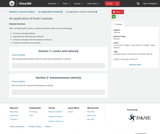Conditional Remix & Share Permitted
CC BY-NC
Rating

After completing this section, students should be able to do the following.Compute average velocity.Approximate instantaneous velocity.Compare average and instantaneous velocity.Compute instantaneous velocity.

Subject:
Calculus
Material Type:
Module
Provider:
Ohio Open Ed Collaborative
06/28/2019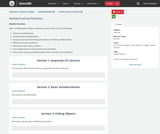Conditional Remix & Share Permitted
CC BY-NC
Rating

After completing this section, students should be able to do the following.Define an antiderivative.Compute basic antiderivatives.Compare and contrast finding derivatives and finding antiderivatives.Define initial value problems.Solve basic initial value problems.Use antiderivatives to solve simple word problems.Discuss the meaning of antiderivatives of the velocity and acceleration.

Subject:
Calculus
Material Type:
Module
Provider:
Ohio Open Ed Collaborative
07/03/2019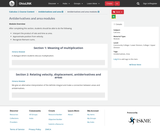Conditional Remix & Share Permitted
CC BY-NC
Rating

After completing this section, students should be able to do the following.Interpert the product of rate and time as area.Approximate position from velocity.Recognize Riemann sums.

Subject:
Calculus
Material Type:
Module
Provider:
Ohio Open Ed Collaborative
07/05/2019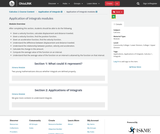Conditional Remix & Share Permitted
CC BY-NC
Rating

After completing this section, students should be able to do the following.Given a velocity function, calculate displacement and distance traveled.Given a velocity function, find the position function.Given an acceleration function, find the velocity function.Understand the difference between displacement and distance traveled.Understand the relationship between position, velocity and acceleration.Calculate the change in the amount.Compute the average value of the function on an interval.Understand that the average value of the function on an interval is attained by the function on that interval.

Subject:
Calculus
Material Type:
Module
Provider:
Ohio Open Ed Collaborative
07/05/2019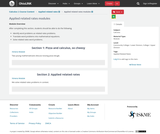Conditional Remix & Share Permitted
CC BY-NC
Rating

After completing this section, students should be able to do the following.Identify word problems as related rates problems.Translate word problems into mathematical equations.Solve related rates word problems.

Subject:
Calculus
Material Type:
Module
Provider:
Ohio Open Ed Collaborative
07/02/2019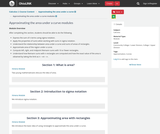Conditional Remix & Share Permitted
CC BY-NC
Rating

After completing this section, students should be able to do the following.Express the sum of n terms using sigma notation.Apply the properties of sums when working with sums in sigma notation.Understand the relationship between area under a curve and sums of areas of rectangles.Approximate area of the region under a curve.Compute left, right, and midpoint Riemann sums with 10 or fewer rectangles.Understand how Riemann sums with n rectangles are computed and how the exact value of the area is obtained by taking the limit as n→∞n→∞ .

Subject:
Calculus
Material Type:
Module
Provider:
Ohio Open Ed Collaborative